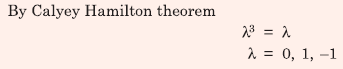# EE GATE 2016 SET 2 - Online Test

Q1. Let f(x) be a real, periodic function satisfying f(−x) = −f(x). The general form of its Fourier series representation would be
Explaination / Solution: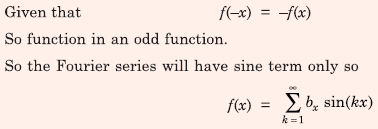Q2. Let x1(t) ↔ X1(ω) and x2(t) ↔ X2(ω) be two signals whose Fourier Transforms are as shown in the figure below. In the figure, h(t) = e-2|t| denotes the impulse response.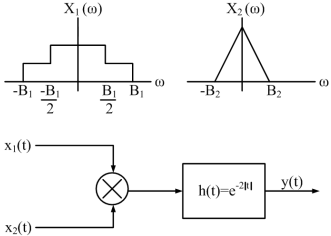For the system shown above, the minimum sampling rate required to sample y(t), so that y(t) can be uniquely reconstructed from its samples, is

Explaination / Solution: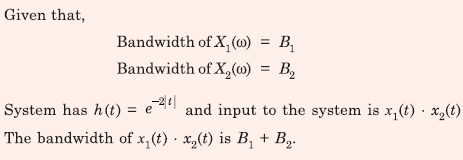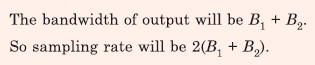Q3. Consider a linear time invariant system x = Ax, with initial condition x(0) at t = 0. Suppose α and β are eigenvectors of (2 x 2) matrix A corresponding to distinct eigenvalues λ1 and λ2 respectively. Then the response x(t) of the system due to initial condition x(0) = α is
Explaination / Solution: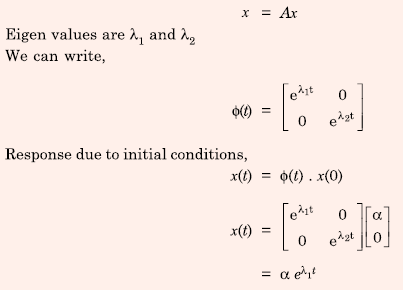Q4. The open loop transfer function of a unity feedback control system is given by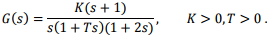The closed loop system will be stable if,
Explaination / Solution: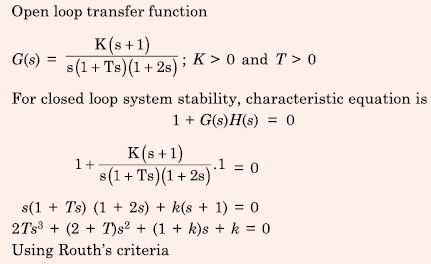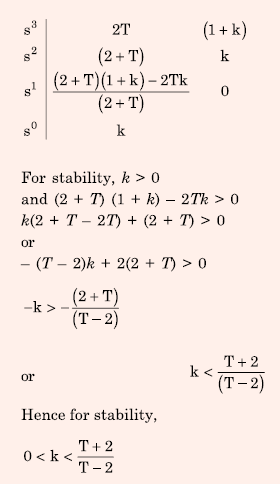Q5. A second-order real system has the following properties:
a) the damping ratio ς = 0.5 and undamped natural frequency ω=10 rad/s
b) the steady state value of the output, to a unit step input, is 1.02.
The transfer function of the system is
Explaination / Solution: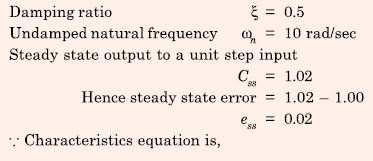Q6. The gain at the breakaway point of the root locus of a unity feedback system with open loop transfer function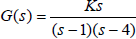is
Explaination / Solution: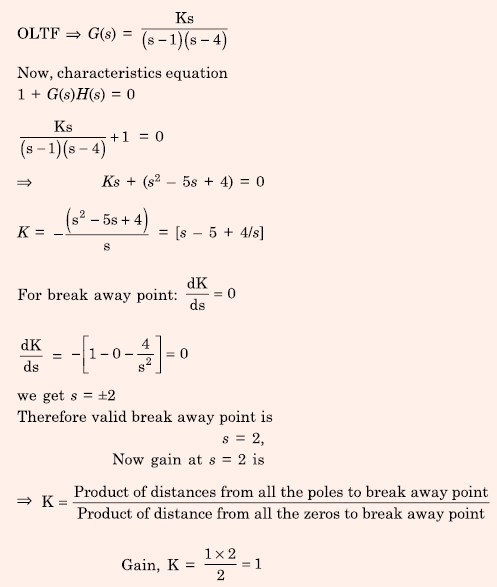Q7. A resistance and a coil are connected in series and supplied from a single phase, 100 V, 50 Hz ac source as shown in the figure below. The rms values of plausible voltages across the resistance (VR) and coil (VC) respectively, in volts, are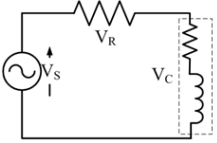Explaination / Solution:Q8. Shaquille O’ Neal is a 60% career free throw shooter, meaning that he successfully makes 60 free throws out of 100 attempts on average. What is the probability that he will successfully make exactly 6 free throws in 10 attempts?
Explaination / Solution: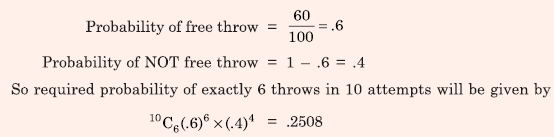Q9. A parallel plate capacitor filled with two dielectrics is shown in the figure below. If the electric field in the region A is 4 kV/cm, the electric field in the region B, in kV/cm, isExplaination / Solution:Q10. A 3 × 3 matrix P is such that, P3 = P. Then the eigenvalues of P are
Explaination / Solution: